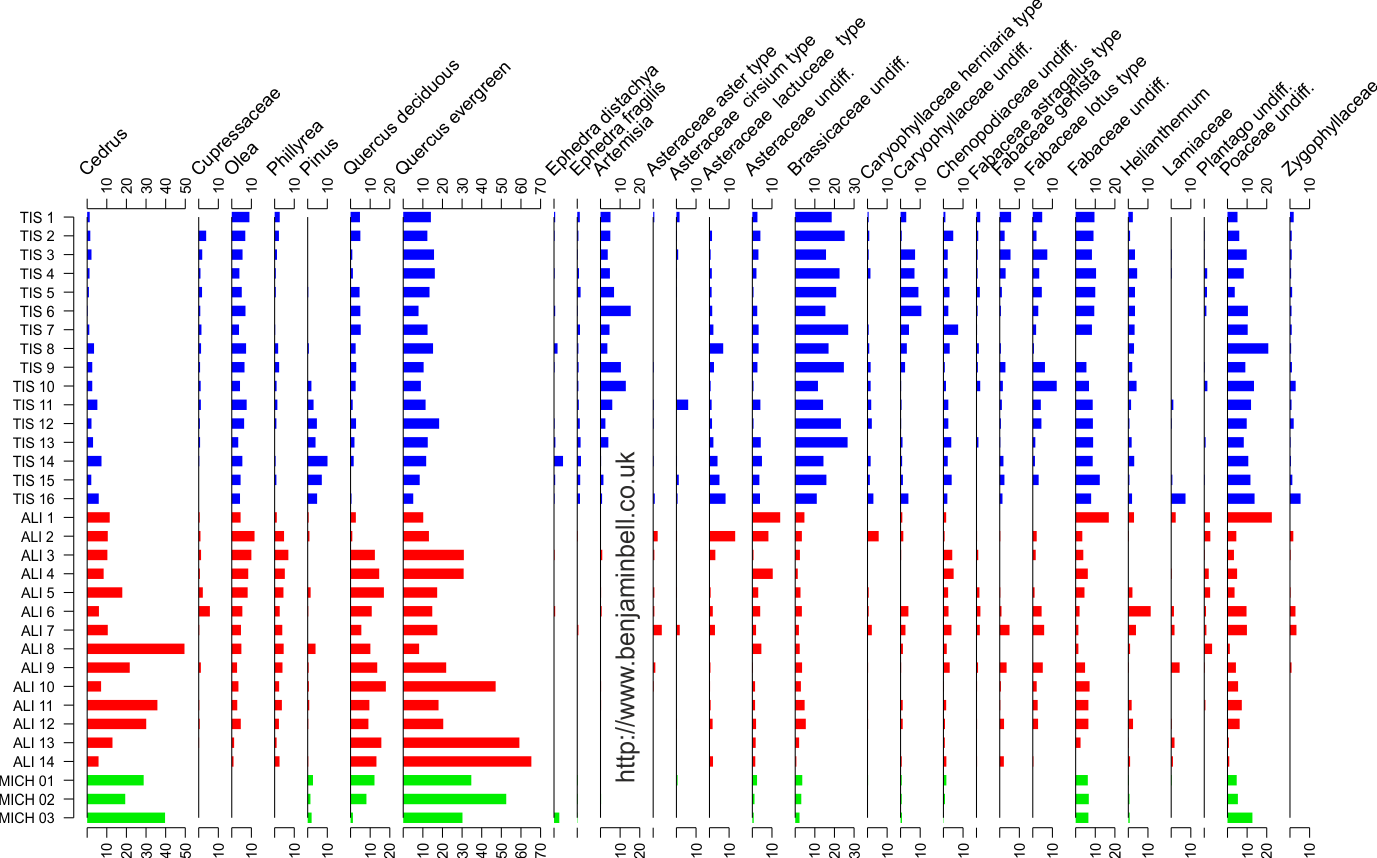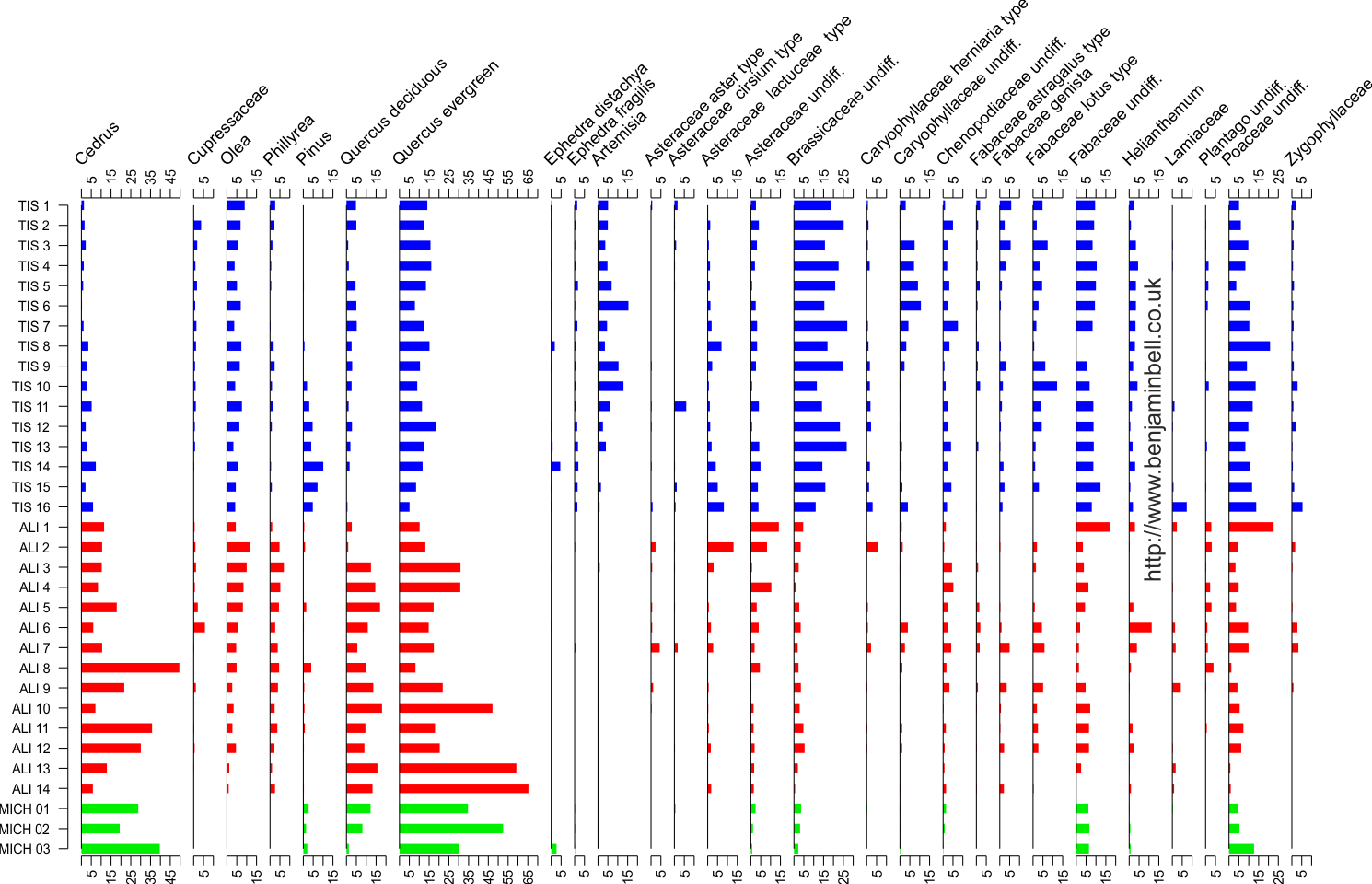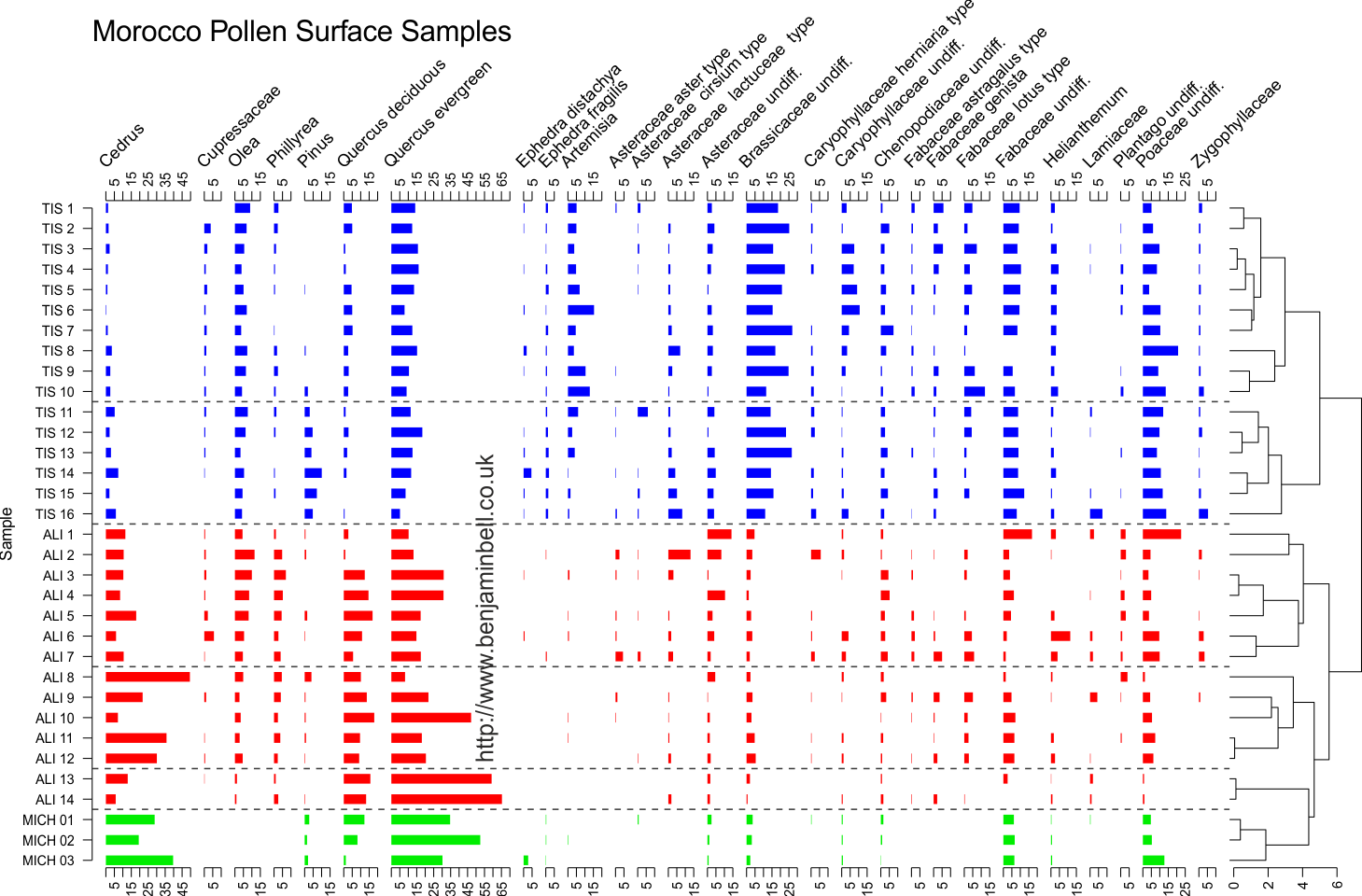## Pages (Mobile)

### Pollen diagrams in R using rioja - Part 2

In the second part of this guide series, I will show you some additional options and "hacks" that you can use for plotting pollen diagrams using the "rioja" package in R.

Click read more for full details, and a step by step guide.

! Never used R before? Check out my getting started with R guide first.

## Guide Information

 Title Pollen diagrams in R using rioja - Part 2 Author Benjamin Bell Published February 07, 2018 Last updated February 16, 2018 R version 3.4.2 Packages base; rioja; vegan Navigation

This guide has been updated:

• Added link to another method to use character strings on the y axis (instead of editing the function).

This is a 3 part guide:

 Part 1: Pollen diagrams using rioja How to plot pollen diagrams using rioja in R. Part 2: Pollen diagrams using rioja "Hacks" to further customise the plot. Part 3: Pollen diagrams using rioja How to use different plot types on the same plot.

## Pollen diagrams using rioja - Part 2

In the second part of this guide, I show you some additional things you can do to your pollen plots. If you missed part 1, you can find it here. Ideally, you need to have read part 1, or you should at least be aware of how to use rioja in R. This guide follows on directly from part 1, and uses the same dataset, so the code below works if you imported and modified the dataset as per part 1.

The great thing about the `strat.plot()` function in rioja, is that it offers lots of options to customise your pollen diagrams. But, there's a few things you can't change on the pollen diagram that you might like to. However, there is a way around this, through editing the source code of the `strat.plot()` function in your R session.

### Change the Y axis labels to characters

Update: For another way to use character strings for the y axis labels that doesn't involve editing the function source code, see part 3 of this guide.

If you remember from part 1, it is not possible to use character strings as the labels for the y axis in the pollen diagram. This is usually not an issue, but if your samples did have character names (such as the surface sample data), then you might want to show this on your plot.

To edit the source code of the function, type `fix(strat.plot)` into the R console. This will start the R text editor, which should contain the source for the function. You can safely edit the source code, as any changes you make will only apply to the R session you are currently working in. i.e. if you close and then re-open R, the source code will revert to the original code.You can use find and replace in this editor to easily find the code that we're going to alter. To change the y axis labels, we need to find the following code:

`labels = as.character(y.tks)` which you should replace with `labels = y.override`

Save your changes, then exit the code editor.

Now, you need to create the "y.override" object, which will contain your sample names, and then run the plot code:

``````library(rioja)
# Create y axis names
y.override <- row.names(ma.pollen1)
# Create colour scheme
p.col.group <- c(rep("blue", times=16), rep("red", times=14), rep("green2", times=3))
# Plot
pol.plot <- strat.plot(ma.pollen1, yvar=y.scale, y.tks=y.scale, y.rev=TRUE, plot.line=FALSE, plot.poly=FALSE, plot.bar=TRUE, col.bar=p.col.group, lwd.bar=10, sep.bar=TRUE, scale.percent=TRUE, xSpace=0.01, x.pc.lab=TRUE, x.pc.omit0=TRUE, srt.xlabel=45, las=2)
``````

Which will result in the following plot:`strat.plot()` will plot the abundance values along the bottom x axis, and the pollen type (or species) along the top x axis in your diagram. If you also wanted to plot the abundance values along the top x axis, you'll need to edit the source code.

Open the source code editor `fix(strat.plot)` and search for the following code:

`axis(side = 1, at = xlabb, labels = xlabbt, mgp = mgpX, cex.axis = cex.axis, ...)`

Leave this code in place and add the following code underneath:

`axis(side = 3, at = xlabb, labels = xlabbt, mgp = mgpX, cex.axis = cex.axis, ...)`

Next, find the following code:

`r <- (usr1 - usr1) * 0.01`

Leave this code in place, but add `+1` after `0.01`, so it should now look like this:

`r <- (usr1 - usr1) * 0.01 +1`

Now, save and exit the code editor. Then run the plot code, adding `mgp=c(3, -0.25, -1)` to your arguments. This will alter the position of the two x axes and labels, moving them closer to the diagram.

``````# Plot
pol.plot <- strat.plot(ma.pollen1, yvar=y.scale, y.tks=y.scale, y.rev=TRUE, plot.line=FALSE, plot.poly=FALSE, plot.bar=TRUE, col.bar=p.col.group, lwd.bar=10, sep.bar=TRUE, scale.percent=TRUE, xSpace=0.01, x.pc.lab=TRUE, x.pc.omit0=TRUE, srt.xlabel=45, las=2, mgp=c(3, -0.25, -1))``````

Which results in the following plot:### More control over X axis labels

`strat.plot()` gives you pretty good control over the x axis labels already, allowing you to change the tick increments, removing the "0" value label, or only showing the min and max labels.

If you wanted more control, for example, showing ticks every 5%, but only showing the labels for every other tick (e.g. 5, 15, 25 etc.), you'll need to edit the source code.

Open the source code editor and find the following code:

`xlabbt <- as.character(xlabb)`

Leave this code in place, and add the following code underneath:

`my.xlabbt <- replace(xlabbt, grep("0", xlabbt), NA)`

Then find `labels = xlabbt` and replace with `labels = my.xlabbt` (There should be two to replace, if you added the top x axis)

Next, run the plot code ensuring it includes `x.pc.inc=5` in the arguments.

``````# Plot
pol.plot <- strat.plot(ma.pollen1, yvar=y.scale, y.tks=y.scale, y.rev=TRUE, plot.line=FALSE, plot.poly=FALSE, plot.bar=TRUE, col.bar=p.col.group, lwd.bar=10, sep.bar=TRUE, scale.percent=TRUE, xSpace=0.01, x.pc.inc=5, x.pc.lab=TRUE, x.pc.omit0=TRUE, srt.xlabel=45, las=2, mgp=c(3, -0.25, -1))``````

Which will result in the following plot: (look closely at the x axis labels to see the changes).### Remove the black lines from the diagram

Surprisingly, `strat.plot()` does not include an option to remove the black lines that are plotted in the pollen diagrams. If you want to remove these lines, or change their appearance, you'll need to edit the source code.

Open the source code editor and find the following code:

`lines(c(0, 0), c(min(yvar, na.rm = TRUE), max(yvar, na.rm = TRUE)), ...)` (note, this code is split over two lines in the editor, try finding: `lines(c(0, 0),` if you get an error)

If you want to remove the lines, then simply delete the code. If you want to change their appearance (e.g. colour, line type etc.), then add the additional variables to the code. Save the code, and exit the editor.

Now, assuming you have made all the changes to the source code listed in this guide, and you were to now plot a pollen diagram (bar plot with cluster analysis), it would look something like this:This is a 3 part guide:

 Part 1: Pollen diagrams using rioja How to plot pollen diagrams using rioja in R. Part 2: Pollen diagrams using rioja "Hacks" to further customise the plot. Part 3: Pollen diagrams using rioja How to use different plot types on the same plot.

Pollen diagrams using rioja - Part 1 - In the first part of this guide, I show you how to plot pollen diagrams using rioja.

Pollen diagrams using rioja - Part 3 - In the third part of this guide, I show you how to use multiple plot types on the same pollen diagram.

1.Hi,
I don't find labels = as.character(y.tks) in fix(strat.plot). Would it be possible to tell me the line number of that code in fix(strat.plott)? Thanks.

1.Hi, i do not know the line number as the R editor in Windows doesn't show them.

But using rioja version 0.9-21, it is definitely there. Use Ctrl-F to find the text in the R code editor - make sure you have clicked somewhere at the very start of the code before using Find.

Best wishes,
Ben

2.Hi Ben,

Thanks for your reply. I have managed to add the species names in the Y axis. But, the some big names do not fit and a portion of such names are shown in the plot. What can I do to show to fit such big species names in the y axis?

Best wishes,
Billah

3.Hi Billah,

I misunderstood your question at first - you want extra space on the Y (vertical) axis for names.

No problem - add the argument "xLeft" to the strat.plot code, and specify a number (this is relative), and the space for the y axis labels should increase.

Here's an example using the code in this guide:

pol.plot <- strat.plot(ma.pollen1, yvar=y.scale, y.tks=y.scale, y.rev=TRUE, plot.line=FALSE, plot.poly=FALSE, plot.bar=TRUE, col.bar=p.col, lwd.bar=10, scale.percent=TRUE, xSpace=0.01, x.pc.lab=TRUE, x.pc.omit0=TRUE, las=2, xLeft=0.2)

Best wishes,
Ben

4.Hi Ben,

Thanks so much for going through this code in so much detail. A question, is it possible to increase the space between the ytks? The reason is that i want to increase the size of the y labels, but doing so shifts causes them to no longer be inline with the ticks of the yaxis

5.Hi - yes, you can specify where the tick marks appear using the y.tks argument. Here's a simple example.

Lets say you have a 1:100 scale, and you want tick marks to appear every 10 - you would specify the ticks as: y.tks=c(0, 10, 20, 30, 40, 50, 60, 70, 80, 90, 100)

If you want less tick marks, you could instead use: y.tks=c(0, 50, 100), which would give you more space.

I hope this is what you were referring to :)

Ben

2.Hi. How can I separate the titles from the graph?

1.Hi,

I am not quite sure what you mean - are you referring to the names of each graph? (e.g. the pollen types).

If you do not want them to appear, add x.names=NA to your plot code.

Best wishes,

3.Hi! Is there a way to add a secondary y-axis? For years bp, por example.

1.Hi,

Take a look at part 3 of this guide. This shows you how to manually control the Y axis.

You can use the same code, to add a secondary y axis to your plot.

However, plotting age and depth can cause problems. Generally, for a depth pollen diagram, you would use a barplot, whereas for age, you would want to use a line graph. This is because in almost all cases age and depth is not a linear 1:1 relationship.

Go ahead and try, and you can see what i mean (e.g. plot the diagram using age and a barplot).

Personally, i think it is best to plot two separate diagrams. However, you can still add age "markers" to a depth diagram, or depth markers to an age diagram - but do this manually in a graphics editor.

Hope that helps,
Ben

4.Hi Ben,

you blog has been extremely helpful! I have a question to do with x-axis limits. Scaling the graphs is not a problem, but on a number of our plots the x-axis is simply not long enough (e.g. the axis extends to 30 but we have abundance data point of 34%). Have been searching for a solution to this but coming up blank. We have tried reducing the space in between plots/ using scale.minmax etc and that doesn't seem to be working either. Is this possible to adapt in strat plot? Thanks in advance! Rebecca

1.Hi Rebecca,

Are you using the argument scale.percent=TRUE? This should change the widths of the x axes, and should extend the axis beyond the highest value.

If you are using scale.percent=FALSE, you can specify "relative" widths using the graph.widths argument - but i am not sure how well this works.

I'm not aware of any other way to control the x axes.

Best wishes,
Ben

5.Hi Ben, thanks for the very helpful guide! I have a question about the y axis if you don't mind. I've replaced the y axis labels with characters, as you describe above, but I'm having trouble getting all my labels onto the axis. I have 63 stratigraphic units, which I know will result in a very long axis/plot, but is fine for my purposes. At the moment it plots every few axis labels. Is there anyway to specify the size of the plot to make it longer so that every label appears? Thanks again for the guide, Emily

1.Hi Emily,

Best wishes,
Ben

6.Hi Ben,
thank you very much for this extremely helpful and detailed blog! My question concerns the very beginning of the plotting of the diagrams. When I plot either the Marocco training set or some of my datasets I always reproduce the same mistake. I get a plot in which no pollen-curves are visible and all the x-axes are clustered together (overlapping each other) over the depth scale. As I get this mistake not only with my different data sets but also when using the Marocco dataset - with simply copy-pasting your first lines of code until the first bar plot - I anticipate that the R version might be responsible? I am using R 4.2.2
Do you have any idea what I could do?
Thanks and best wishes,
Sascha

1.Hi Sascha,

Thats a strange result! And I'm not entirely sure what could be causing it. The later r version shouldn't matter.

If you could send me the code you are using, I'll be happy to take a look. My email is on the About me page.

Best wishes,
Ben

Comments are moderated. There may be a delay until your comment appears.